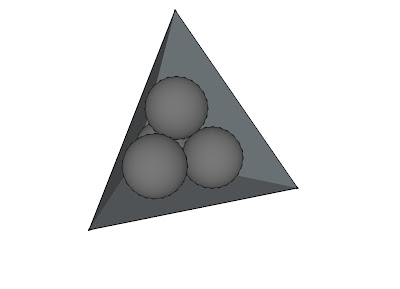## Monday, December 5, 2011

### Beautiful Mathematics

Martin J. Erickson, Truman State UniversityWhat is your favorite mathematical concept? Is it a theorem that you especially like, or is it a proof that you’ve always remembered? Perhaps it’s a challenging problem that you were able to solve. Or a mathematical construction, or a particular mathematical object.

People’s mathematical tastes vary widely. Some enjoy applications of math to real-world problems. Others revel in math’s abstractions. Some like problem-solving, others theory-building. Whatever your mathematical tastes, you have certain thoughts about what constitutes mathematical beauty.

I wrote the book Beautiful Mathematics (MAA, 2011) to share mathematical concepts and creations that some people consider beautiful. I organized the book into chapters covering mathematical words, images, formulas, theorems, proofs, solutions, and unsolved problems. There’s also coverage of mathematical foundations and a set of exercises (with solutions) covering the topics raised in the text.

My hope is that this material will inspire students and teachers. One of the best motivations to learn mathematics is that math can be beautiful.

Here is a sample topic from the book. It’s a simply stated problem involving a tetrahedron and four spheres. Can you solve it?

Four spheres of unit radius are contained in a regular tetrahedron in such a way that each is tangent to three faces of the tetrahedron and to the other three spheres. What is the side length of the tetrahedron?What is your solution? Try to make your proof as simple and elegant as possible.

#### 1 comment:

1.Cute. I make it $2(1+\sqrt 6)$. Consider the tetrahedron whose vertices are the centres of the spheres. It has edge length 2. By a standard formula, it's inradius is therefore $1/\sqrt 6$. The base of the large tetrahedron is distance 1 further from the centroid of everything than the base of the small one, and so the large one is larger by a factor $1+1/\sqrt 6\over 1/\sqrt 6 = 1+\sqrt 6$.